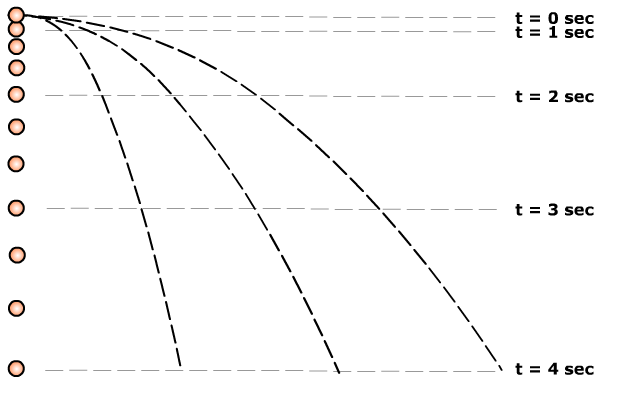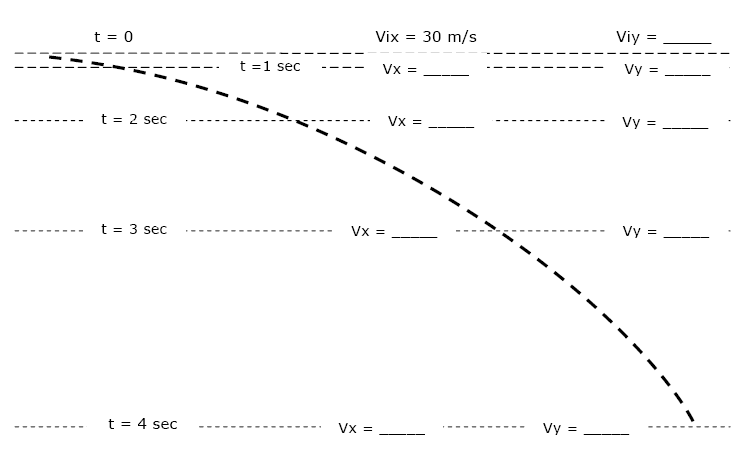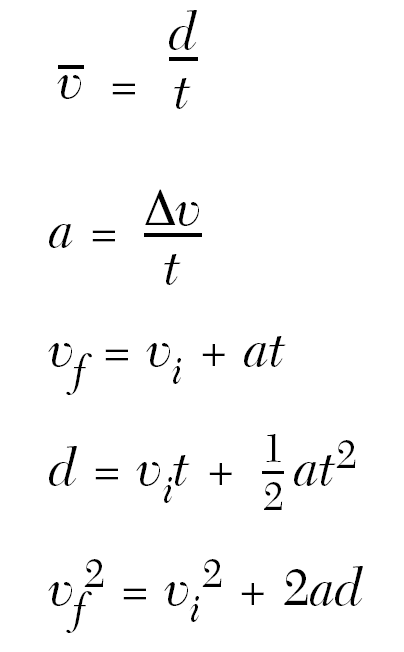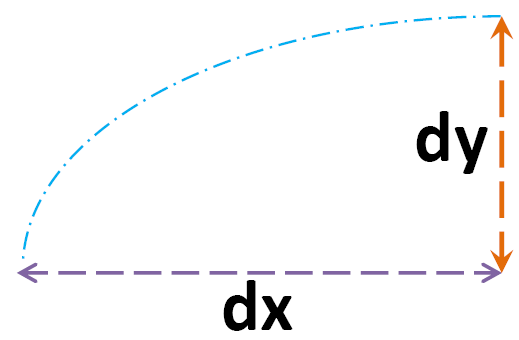Show Me The Physics

Object Thrown Horizontally

[ Flash ]

How many velocities?

[ Flash ]

2 velocities

Horizontal velocity - Determined by thrower

Vertical velocity - ??????

acceleration due to gravity

b. Objects propelled horizontally- have 2 velocities Vx, Vy

- Vx is determined by thrower

- Vx  constant  (neglect fricition)

- Viy = 0 (initial vert. vel.)

- Vy changed by ........

force of gravity

Ex) Object thrown horizontally a velocity of 4 m/s

 time (sec) Vx (m/s) Vy (m/s) 0 4 0 1 4 10 2 4 20 3 4 30

Object dropped/Objects thrown horizontallySame:

a) ay  (+10 m/s2 )

b) vertical drop -  dy

c) time in air

d) same Vy

Compare each sphere's:

A) height above the ground,

B) time in the air

| Flash |
Show Me The Physics Website

Compare the time it takes for the marbles to hit the ground.Dropped/Projected Horizontally
Rutgers U[ Flash ]
Show Me The Physics WebsiteEx1) A rock is thrown horizontally from the top of a cliff @ 150 m/s.

a) How long does it take the rock to fall 45 m vertically.

Step 1 - Underline

Ex1) A rock is thrown horizontally from the top of a cliff @ 150 m/s

a) How long does it take the rock to fall 45 m vertically.

Step 2 - Turn information into letters

(Don't mix y information with x information)

 Horizontal Vertical Vx = 150 m/s Viy = 0 (thrown horizontally) t = ? ay=+9.8 m/s2 dy = 45 m t = ?

Step 3 -  Substitute and Solve∆dy = Viyt + 1/2ayt2

45 m = 0 + ½(9.8 m/s2)t2

45 m = 4.9t2

9 = t2

t = 3 secondsA rock is thrown horizontally from the top of a cliff @ 150 m/s

b) Find the rock's vertical velocity at 45 meters

 Horizontal Info Vertical Info Vx = 150 m/s Viy = 0 (thrown horizontally) ay=+9.8 m/s2 dy = 45 m Vfy = ?Vfy2 = 0+ 2(+9.8 m/s2)45m

Vfy2 = 19.6(45)

Vfy = 30 m/s

Only X Equation

** dx = Vxt **

(from Vx = dx/t)dx - Horizontal distance
Vx - horizontal velocity

ORIGINAL QUESTION

Ex1) A rock is thrown horizontally from the top of a cliff @ 150m/sHow long does it take the rock to fall 45 m vertically

c) What was the rocks' horizontal displacement after 3.0 seconds?

 Horizontal Vertical Vx = 150 m/s Viy = 0 (thrown horizontally) t = 3.0 sec ay = 9.8 m/s2 dx = ? dy = 45 m t = 3.0 sec

Given

Vx = 150 m/s

(Object thrown horizontally)

dx = ?

(A.K.A. Range)

t = 3.0 seconds

** dx  = Vxt **

dx = 150 m/s(3.0 seconds)

= 450 mProjectile Fired Horizontally 2